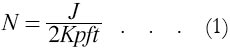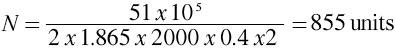# Energy Stored in Capacitors

(Reproduced from "Zero to Eighty" pp.323 by EF Northrup)

A certain type of commercial capacitor (condenser) is rated at 11.7 microfarads and is intended for use on 1000-cycle, 1250-volt circuits. The maximum electrostatic energy stored per half cycle in this capacitor is equivalent to 1.865 kilogram meters. Calling this quantity K, we find the following general equation for the number of capacitor units for use in the electric gun:where N is the number of capacitor units, J the requisite kinetic energy in kilogram meters, K the equivalent kilogram meters per unit per half cycle, p the efficiency of the electric gun in producing motion, f the frequency in cycles per second, and t the time in seconds necessary for the projectile to acquire the desired velocity.

For the Utah gun the value of N was found by assigning the following values to the factors in equation (1):
J = 51 x 105, K = 1.865, p = 0.4 (40% efficiency), f = 2000, t = 2. Substituting in (1):< Previous Page 11 of 17 Next >